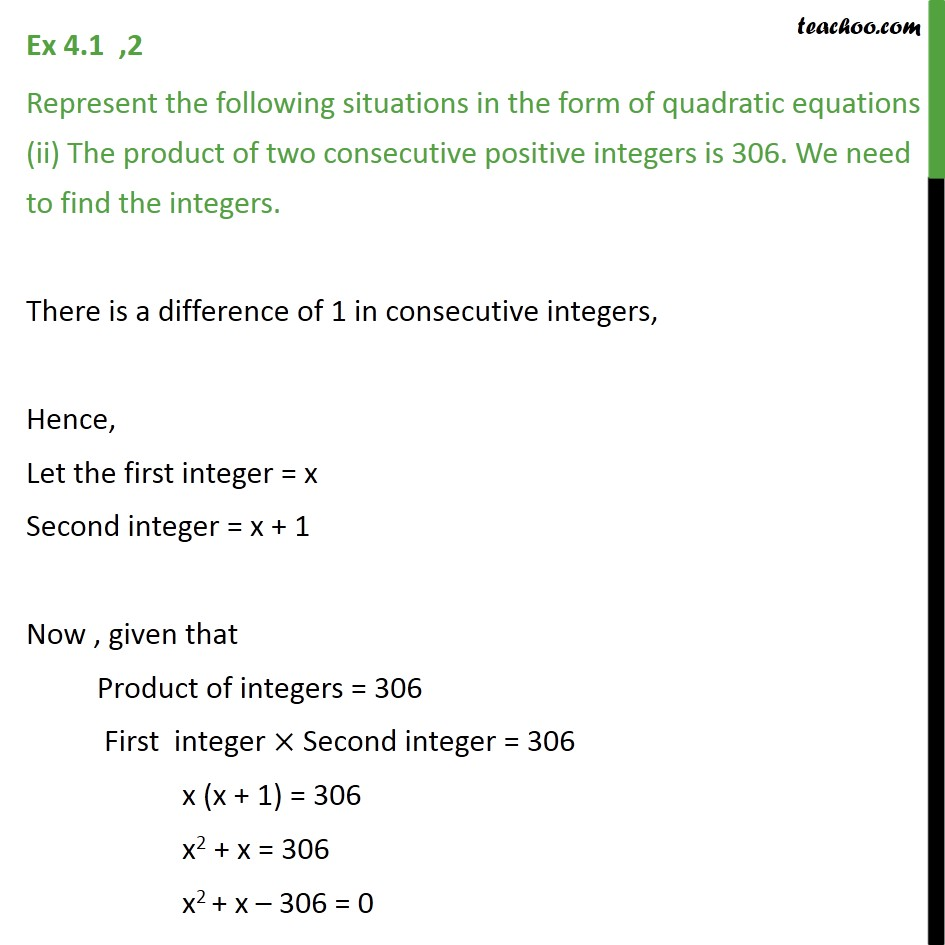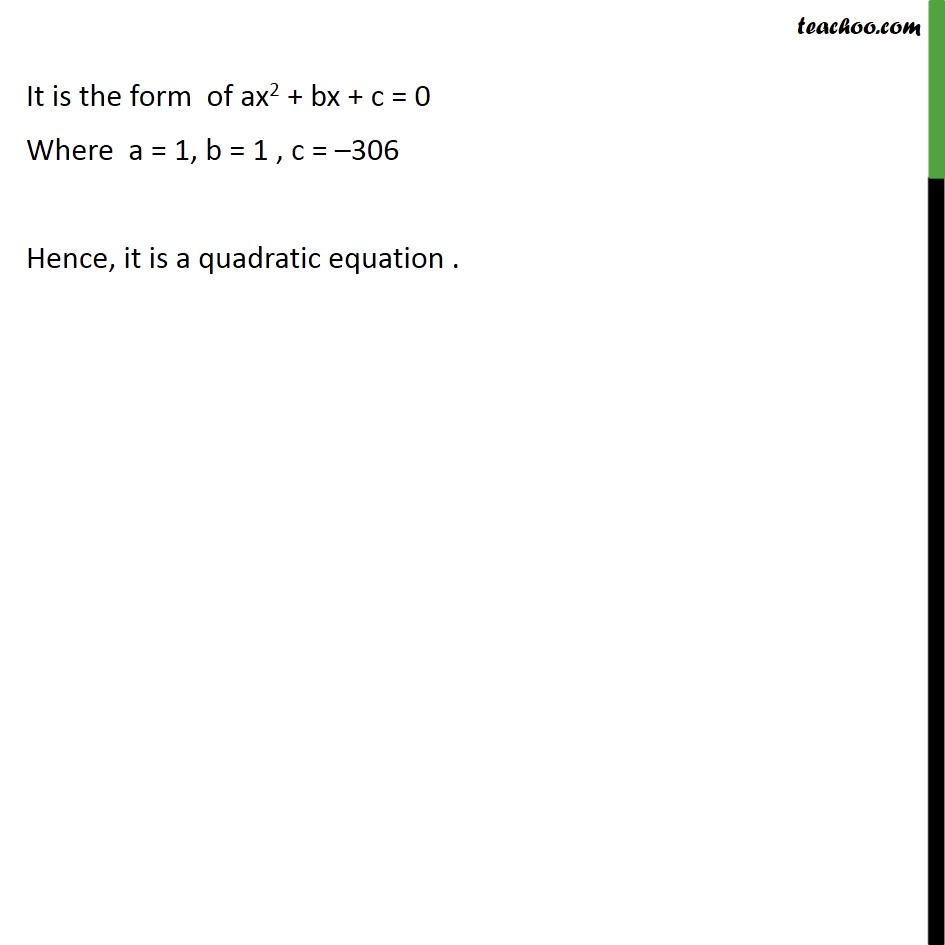Ex 4.1

Chapter 4 Class 10 Quadratic Equations
Serial order wiseLearn in your speed, with individual attention - Teachoo Maths 1-on-1 Class

### Transcript

Ex 4.1 ,2 Represent the following situations in the form of quadratic equations : (ii) The product of two consecutive positive integers is 306. We need to find the integers. There is a difference of 1 in consecutive integers, Hence, Let the first integer = x Second integer = x + 1 Now , given that Product of integers = 306 First integer Second integer = 306 x (x + 1) = 306 x2 + x = 306 x2 + x 306 = 0 It is the form of ax2 + bx + c = 0 Where a = 1, b = 1 , c = 306 Hence, it is a quadratic equation .